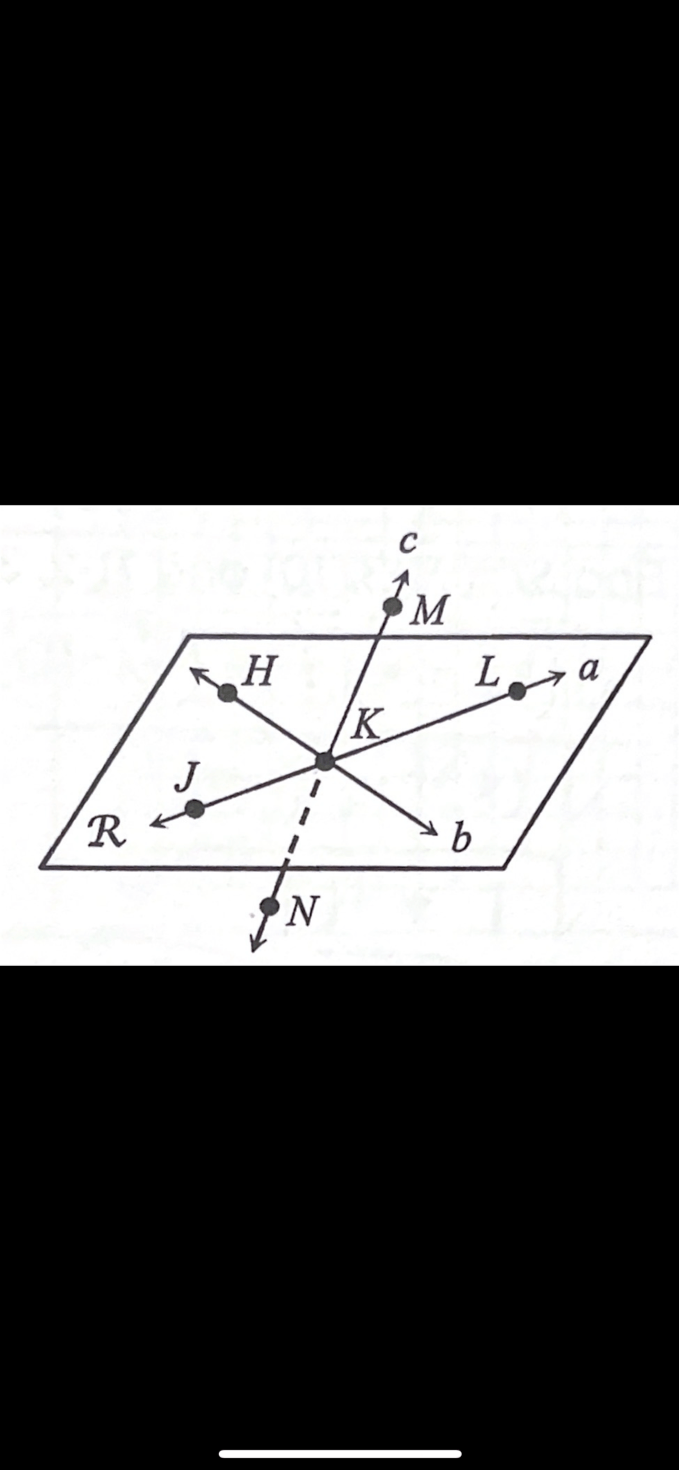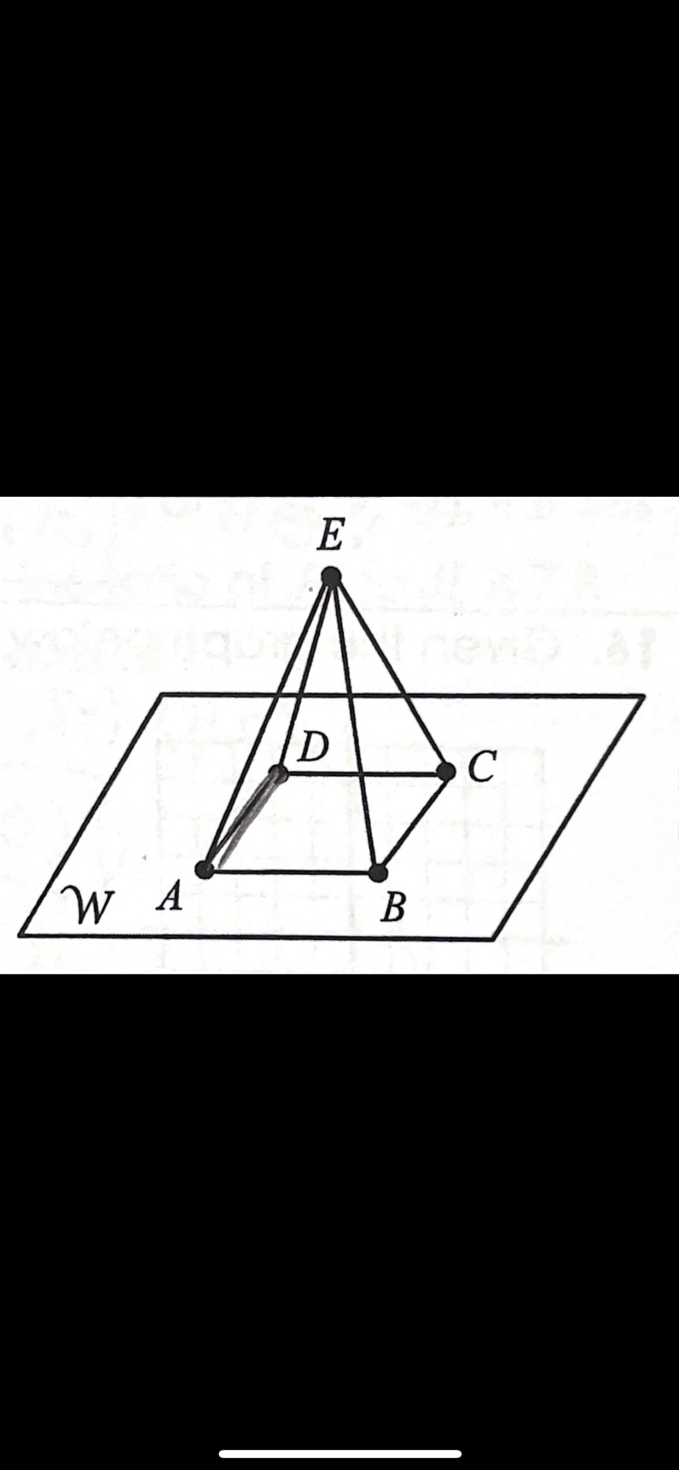Vocabulary
Points, Lines, & Planes
Distance and Midpoint
Angle Measures
100

An ____ is an intersection of rays at an endpoint.

Angle

100

Name 2 points collinear to point K and name one point non-coplanar to plane R.M,N or J,L

N or M

100

If DF=42, DE=(7x+1), and EF=(4x-3), find DE.

DE=29

100

What are the formulas for Midpoint and Distance?

((x+x)/2, (y+y)/2)

√(x_2-x_1)^2+(y_2-y_1)^2

100

If m<DEF=117, m<DEG=(12x+1), and m<GEF=(5x-3), find the value of x.

x=7

200

What are complementary angles?

200

Give another name for line b.<-->HK

200

If JL=(10x-2), JK=(5x-8), and KL=(7x-12), find KL.

KL=51

200

Find ST if S(-3,10) and T(-2,3).

d~7.1

200

If m<PQS=16º, m<SQR=(9x+17), and m<PQR=(12x-6), find m<PQR.

m<PQR=150º

300

What are supplementary angles?

300

How many planes are shown in the figure?5

300

If S is the midpoint of RT, RS=(5x+17), and ST=(8x-31), find RS.

RS=97

300

Find BC if B(8,-7) and C(-4,-2).

d=13
300

<1 and <2 form a linear pair. If m<1=(18x-1) and the m<2=(23x+17), find m<2.

m<2=109º

400

What does a bisector do?

Specifically, what does a perpendicular bisector do?

Divides an angle, line, or segment into 2 congruent parts.

Divides a segment perpendicular to its midpoint.

400

Give another name for plane W.Plane ABC, etc.

400

If line y bisects AC, AB=(4-5x) and BC=(2x+25), find AC.

AC=38

400

Find the coordinates of the midpoint of HK if H(-1,2) and K(-7,-4).

M=(-4,-1)

400

<1 and <2 are vertical angles. If m<1=(5x+12) and m<2=(6x-11), find m<1.

m<1=127º

500

____ are adjacent angles that are supplementary. Combined, they form a straight line.

Linear Pair

500

Name the intersection of Plane ADE and plane W.500

If B is the midpoint of AC, AC=CD, AB=3x+4, AC=11x-17, and CE=49, find DE.

DE=11

500

Find the coordinates of Z if Y is the midpoint of XZ, X(-10,9), and Y(-4,8).

Z(2,7)

500

The measure of <P is five less than four times the measure of <Q. If <P and <Q are supplementary angles, find m<P.

m<P=143º

Click to zoom